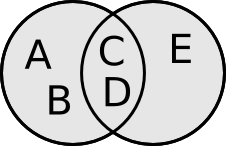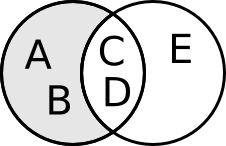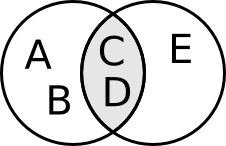## Set operations on ruby arrays

Ruby provides some very interesting set operations on arrays.

given the two arrays A and B wich look like this

``````A = ["A", "B", "C", "D"]
B = ["C", "D", "E"]
``````

There are three set operations we can use that union, intersection and difference.

## Union``````A | B
``````

contains all elements from both sets without doublicates. So this results in ["A", "B", "C", "D", "E"]

## Difference``````A - B
``````

contains all elements from set A that are not in set B. So this results in ["A", "B"]

## Intersection``````A & B
``````

contains all elements that are in set A and in set B. So this results in ["C", "D"]